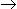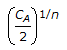# Chemical Engineering - Chemical Reaction Engineering

Exercise : Chemical Reaction Engineering - Section 4
1.
Which of the following resistances is not involved in a gas phase catalytic (gas-solid) reaction ?
Ash resistance.
Gas film and pore surface diffusion resistances for reactants.
Surface phenomenon resistance.
Gas film and pore surface diffusion resistances for products.
Explanation:
No answer description is available. Let's discuss.

2.
If the time required to change the concentration of reactant to half its original value is independent of the initial concentration, the order of reaction is
zero
one
two
three
Explanation:
No answer description is available. Let's discuss.

3.
A batch adiabatic reactor at an initial temperature of 373°K is being used for the reaction, AB. Assume the heat of reaction is - 1kJ/mole at 373°K and heat capacity of both A and B to be constant and equal to 50J/mole.K. The temperature rise after a conversion of 0.5 will be
5°C
10°C
20°C
100°C
Explanation:
No answer description is available. Let's discuss.

4.
If CA is the quantity of reactants initially present, the quantity left after 'n' half periods will be equal to(CA)1/2n